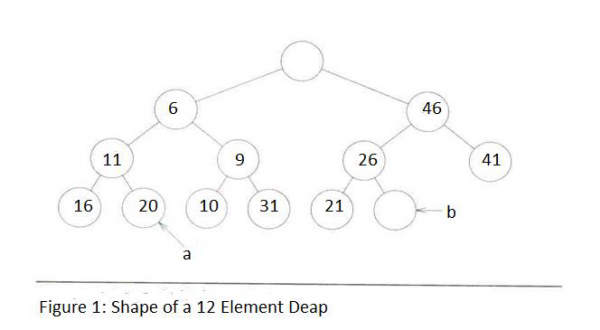# Deaps in Data Structure

Data Structure AlgorithmsAnalysis of AlgorithmsAlgorithms

Deap is defined as a data structure which has no element or key value at the root node. It is formed by implementing the following rules −

• There is no element at root node that indicates root node is empty.
• Left subtree of the deap shall indicate min heap.
• Right subtree of deap shall indicate max heap.

Thus, correctness to the following statement can be provided mathematically by a deap structure −

If the left sub tree and right sub tree of certain nodes are non-empty, and their corresponding nodes can be represented by ‘a’ and ‘b’ respectively, then −a.KeyValue <= b.KeyValue